# 深度学习之长短期记忆网络LSTM钱魏Way · · 6 次浏览

## 普通RNN存在的问题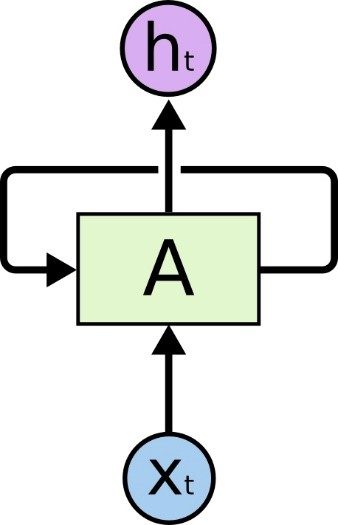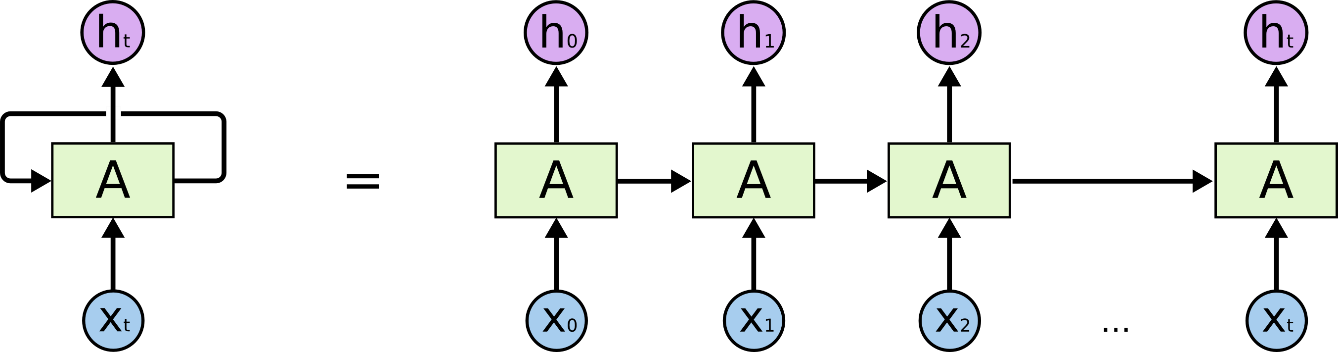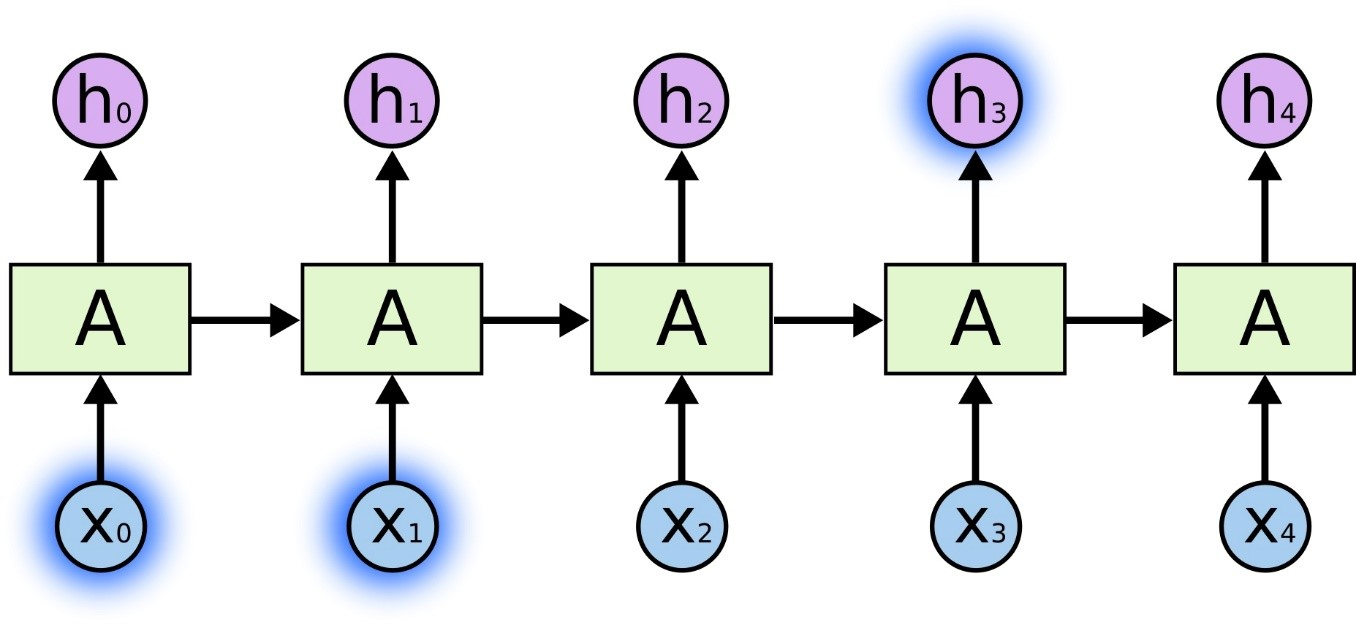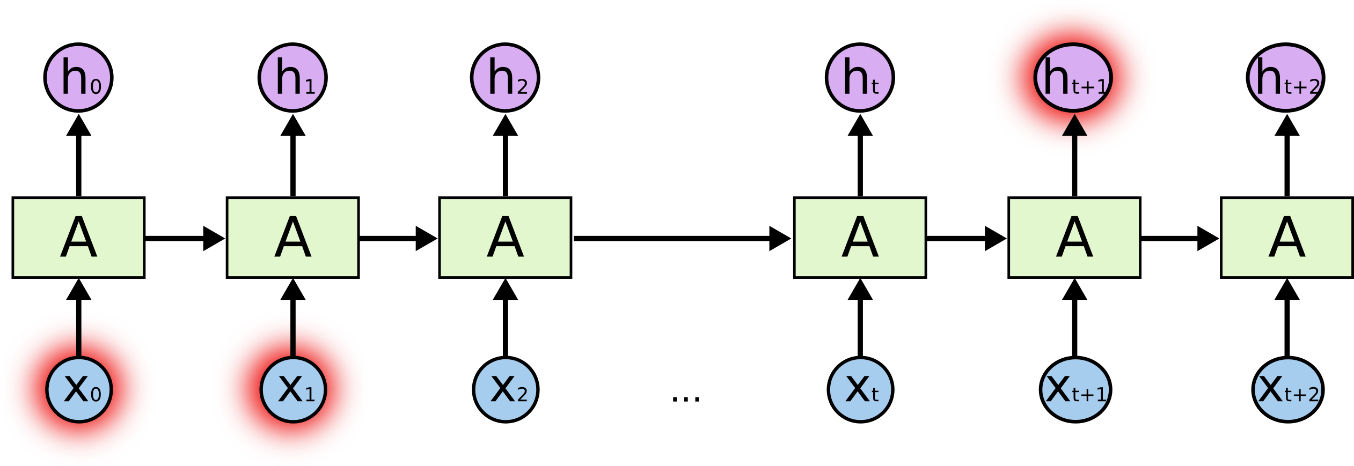## LSTM网络简介

LSTM，全称为长短期记忆网络(Long Short Term Memory networks)，是一种特殊的RNN。LSTM由Hochreiter & Schmidhuber (1997)提出，许多研究者进行了一系列的工作对其改进并使之发扬光大。LSTM在许多问题上效果非常好，现在被广泛使用。

LSTM在设计上明确地避免了长期依赖的问题。所有的循环神经网络都有着重复的神经网络模块形成链的形式。在普通的RNN中，重复模块结构非常简单，例如只有一个tanh层。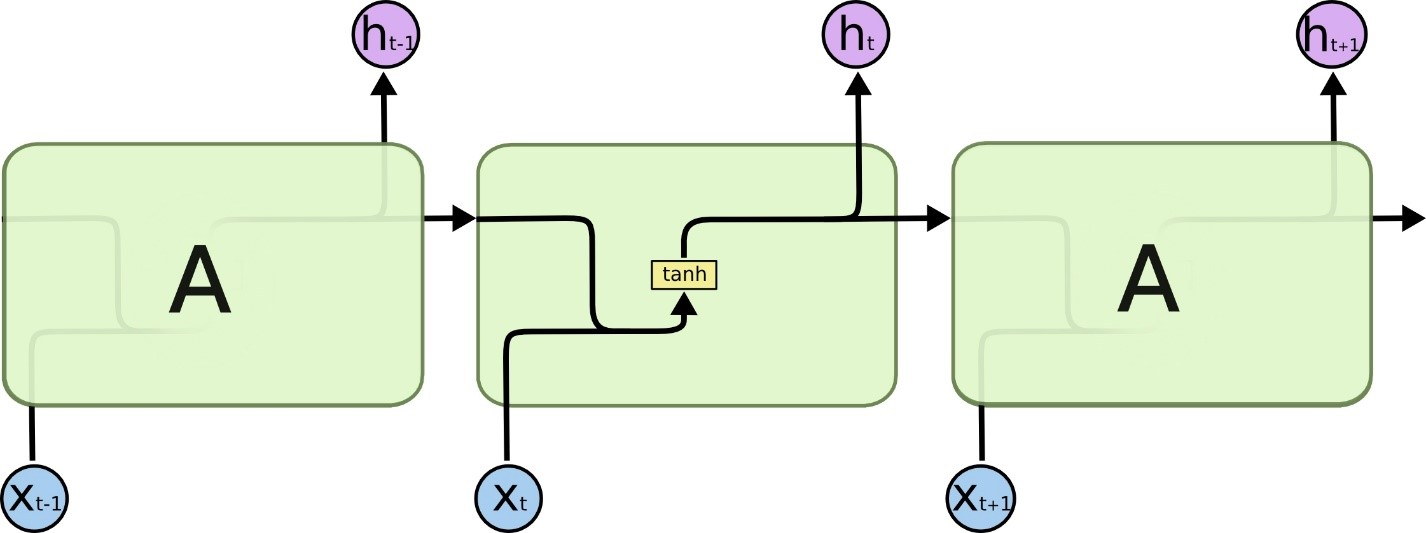LSTM也有这种链状结构，不过其重复模块的结构不同。LSTM的重复模块中有4个神经网络层，并且他们之间的交互非常特别。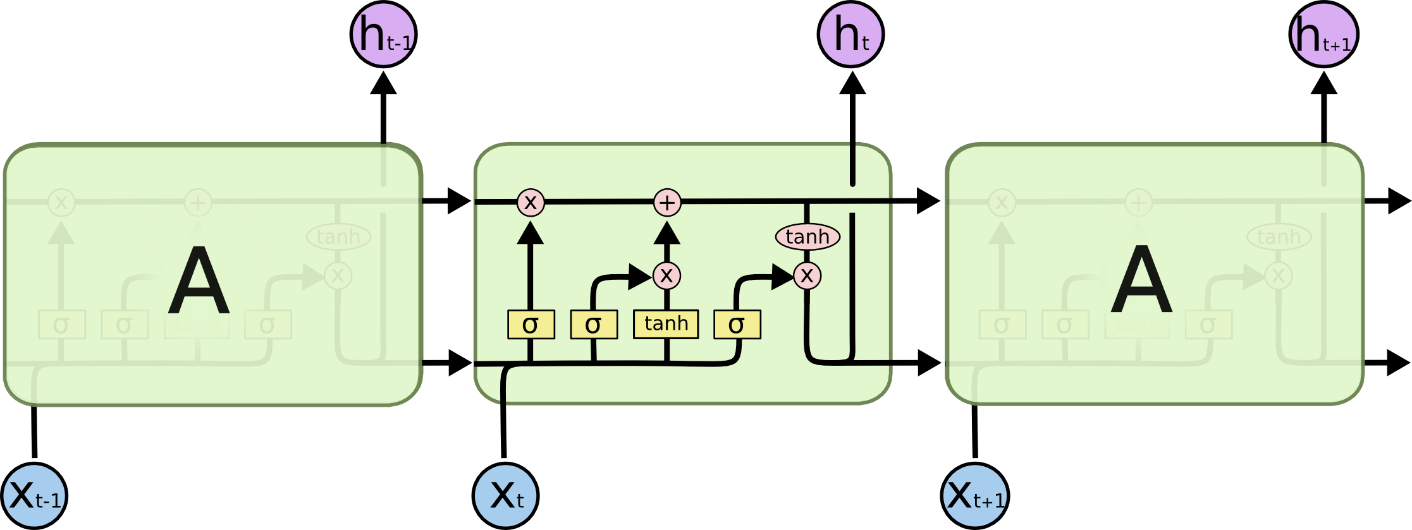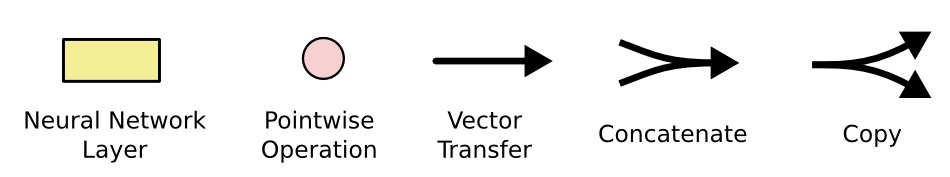## LSTM网络的实现原理

LSTM的主要思想是采用一个叫做“细胞状态(state)”的通道来贯穿整个时间序列。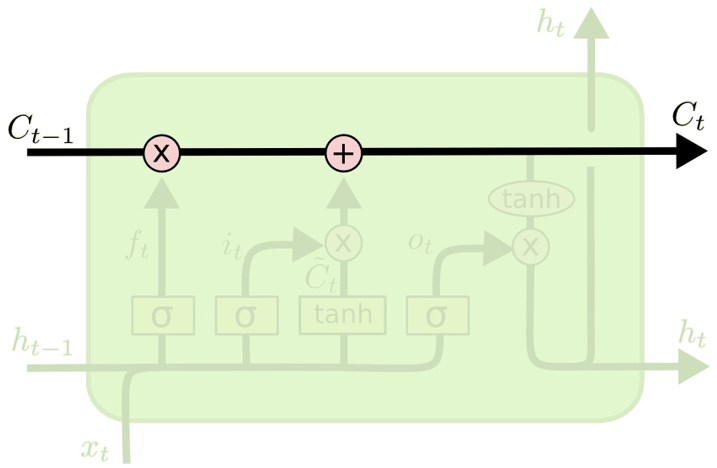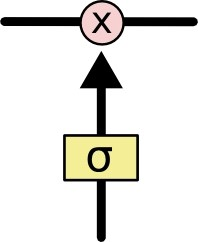Sigmoid层输出0~1之间的值，每个值表示对应的部分信息是否应该通过。0值表示不允许信息通过，1值表示让所有信息通过。一个LSTM有3个这种门，来保护和控制元胞状态。

### 遗忘门

“遗忘门”决定之前状态中的信息有多少应该舍弃。它会读取 $h_{t-1}$ 和 $x_t$的内容,$\sigma$符号代表Sigmoid函数，它会输出一个0到1之间的值。其中0代表舍弃之前细胞状态$C_{t-1}$中的内容，1代表完全保留之前细胞状态$C_{t-1}$中的内容。0、1之间的值代表部分保留之前细胞状态$C_{t-1}$中的内容。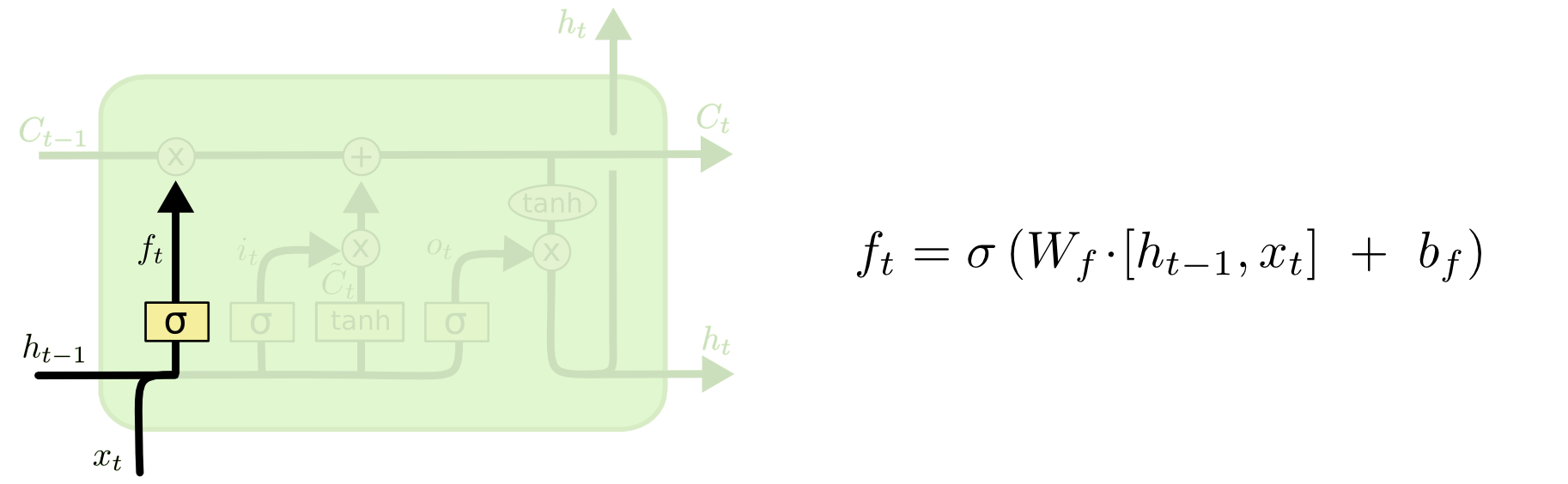### 输入门

“输入门”决定什么样的信息保留在细胞状态$C_t$中，它会读取 $h_{t-1}$ 和 $x_t$的内容,$\sigma$符号代表Sigmoid函数，它会输出一个0到1之间的值。和“输入门”配合的还有另外一部分，即下图中计算tanh层的部分，这部分输入也是$h_{t-1}$ 和 $x_t$，不过采用tanh激活函数，将这部分标记为$\tilde c^{(t)}$，称作为“候选状态”。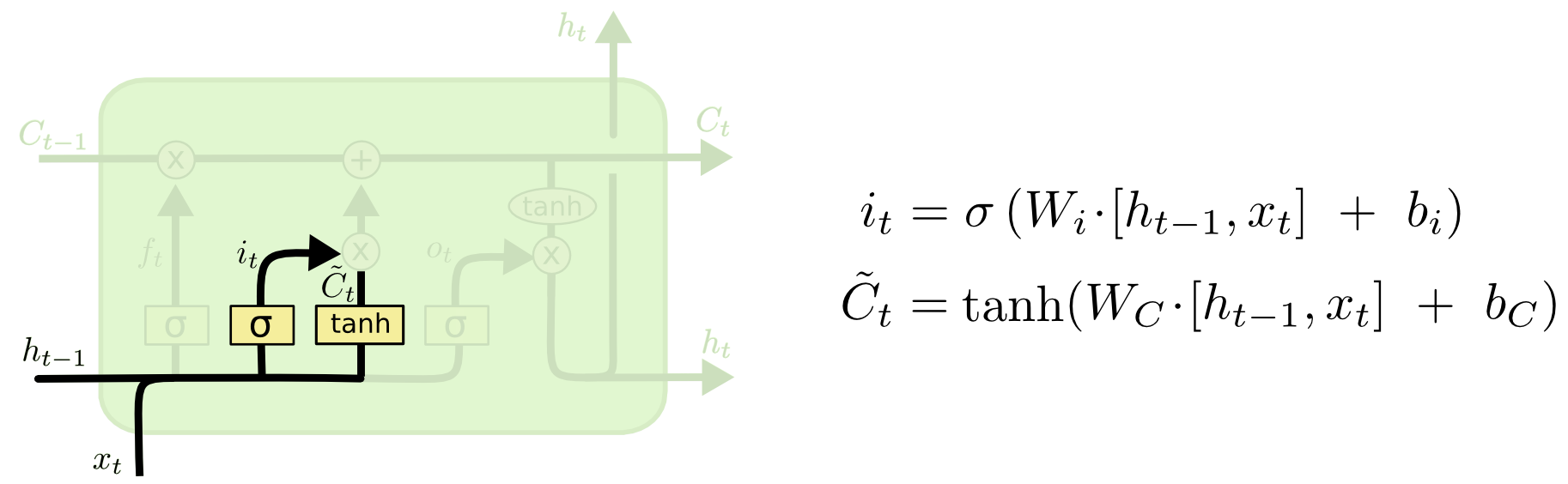### 细胞状态更新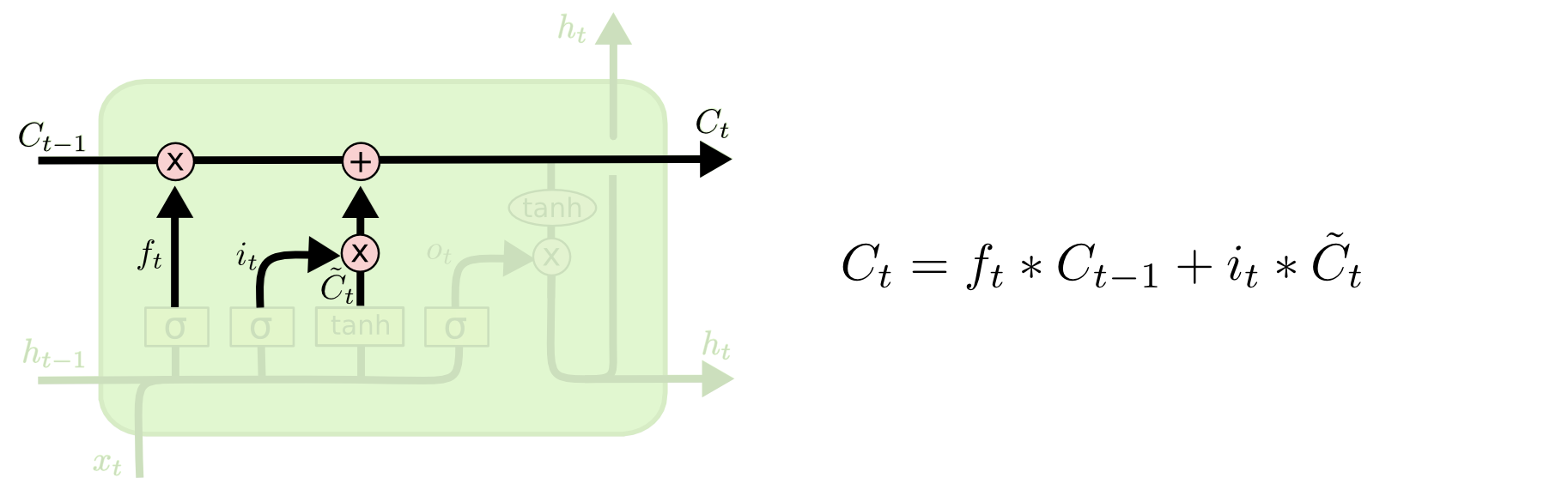### 输出门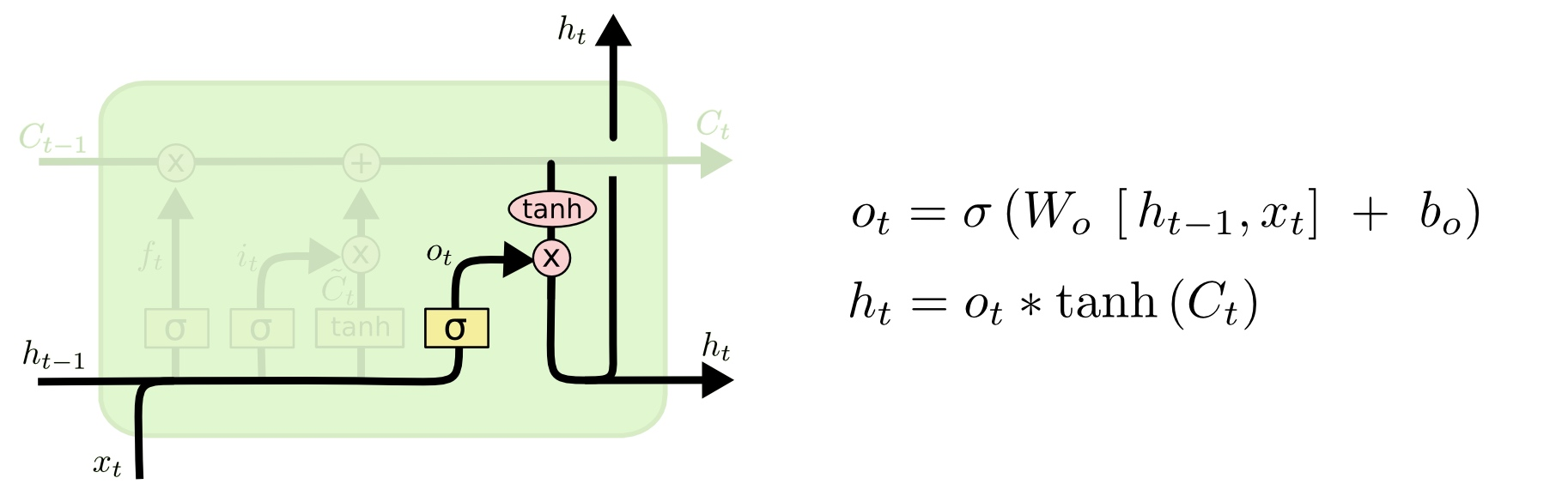## 使用LSTM对IMDB评论进行情感分析

from keras.datasets import imdb
from keras.layers import LSTM, Dense, Embedding
from keras.models import Sequential
from keras.preprocessing import sequence

max_features = 20000
# cut texts after this number of words (among top max_features most common words)
maxlen = 80
batch_size = 32

(x_train, y_train), (x_test, y_test) = imdb.load_data(num_words=max_features)
print(len(x_train), "train sequences")
print(len(x_test), "test sequences")

print("x_train shape:", x_train.shape)
print("x_test shape:", x_test.shape)

print("Build model...")
model = Sequential()

# try using different optimizers and different optimizer configs

print("Train...")
model.fit(
x_train, y_train, batch_size=batch_size, epochs=15, validation_data=(x_test, y_test)
)
score, acc = model.evaluate(x_test, y_test, batch_size=batch_size)
print("Test score:", score)
print("Test accuracy:", acc)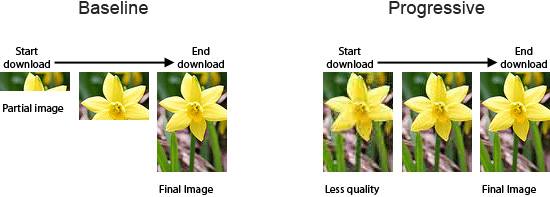##### 使用渐进式JPEG来提升用户体验##### 网站开发图片格式对比与选择